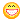Physics.ray Strange Issues (inc. video) Author Message
fatcoderMember

 Post: #1 Physics.ray Strange Issues (inc. video) To demonstrate the problem, I have modified the "Detecting objects under cursor" tutorial. I made two changes to the tutorial code. If you want to try it for yourself, then change the code in the Init method so it creates 10 boxes like this. Code: ```REPA(obj)    obj[i].create(Box(RandomF(0.1f, 0.5f),Random(Box(10, 1, 10)))).group(GROUP_OBJ);``` Then add the following code to the end of the draw() method. Code: ```for(Flt x = -5; x < 5; x += 0.1f) for(Flt z = -5; z < 5; z += 0.1f) {    if(Physics.ray(Vec( x, 5.0f, z), Vec(x, -5.0f, z), &phys_hit, IndexToFlag(GROUP_OBJ)))       D.dot(RED, Vec(x, -1.5f, z)); }``` Video 1 displays the first problem using the code above. Video 1 As you can see, the grid of rays stop hiting the objects as they fall to the ground, even though the rays are passing right through the objects. Compare the ray start and end position with the object positions and sizes. What is the problem here? Why do the rays stop hitting the objects??? Now if we reverse the rays like this (note the start and end of the rays are reversed). Code: ```for(Flt x = -5; x < 5; x += 0.1f) for(Flt z = -5; z < 5; z += 0.1f) {    if(Physics.ray(Vec(x, -5.0f, z), Vec(x, 5.0f, z), &phys_hit, IndexToFlag(GROUP_OBJ)))       D.dot(RED, Vec(x, -1.5f, z)); }``` Video 2 displays the second problem using the changed code above. Video 2 As you can see, the objects are now being hit by the rays all the time. I don't understand why reversing the direction of the rays should make any difference. However, this better shows the second problem (which happens no matter which way the rays are directed). Notice how the red dots (which is where a ray has "hit" an object) do not line up with any of the boxes. What is going on here? If I draw the actual hit position (i.e. phys_hit.plane.pos) then the dots appear in the correct places. Why doesn't phys_hit.plane.pos.xz() == Vec2(x,z)??? In fact phys_hit.plane.pos.xz is always exactly double Vec2(x,z). Why is this? For example, if you fire a ray from (2,5,4) to (2,-5,4) and it hits an object at 0.0f on the ground plane... the resulting phys_hit.plane.pos = (4,0,8)?!?!?! How can I fire a ray through the scene in one place and have the result return back a location exactly 2x the distance away from where the ray passed through the scene??? Is there a bug here? P.S. add this to the update method if you need to spin around the scene to view it better. Code: `CamHandle(0.1f, 10, CAMH_ZOOM|(Ms.b(1)?CAMH_MOVE:CAMH_ROT));`
02-21-2012 12:51 PM
ZervoxMemberPost: #2 RE: Physics.ray Strange Issues (inc. video) Code: ```for(Flt x = -5; x < 5; x += 0.1f) {   for(Flt z = -5; z < 5; z += 0.1f) {    PhysHit phys_hit;   if(Physics.ray(Vec( x, 5.0f, z), Vec(0,-1.0f,0)*100, &phys_hit, IndexToFlag(GROUP_OBJ)))    D.dot(RED, Vec(x, -1.5f, z)); } }``` Phys ray = Vec starting pos, position*in that direction, I don't know if phys ray hits from the inside out but it seems not so that might be the case? the move vector in Physics.ray = Dir*length in this case straight down 100 meter
02-21-2012 02:00 PM
fatcoderMember

 Post: #3 RE: Physics.ray Strange Issues (inc. video) Thanks Zervox for making me feel like a fool! I have used Phyics.ray a million times without a problem until today I just couldn't figure out what was going wrong. I completely forgot that it is start position and direction... not start and end position... I just drew a blank! I'm getting back to work with my tail between my legs02-21-2012 02:17 PM
fatcoderMember

 Post: #4 RE: Physics.ray Strange Issues (inc. video) I have realised why I got confused before. I have been working a lot with Pathworld.ray, which takes a start and an end Vec. Then I went back to do something with Physics.ray, which takes a start and a move Vec. Very easy to forget what to pass when they both work differently. Esenthel, if possible, perhaps you should changed Pathworld.ray to take a start and a move Vec just like Physics.ray to keep them working the same way.
02-23-2012 10:53 AM
 « Next Oldest | Next Newest »